# Learn Python by Building a Cryptocurrency AnalysisCryptocurrency Analysis with Python. In this part, I am going to analyze which coin (Bitcoin, Ethereum or Litecoin) was the most profitable in the last two months using buy and hold strategy. ... First, let’s download hourly data for BTC, ETH, and LTC from Coinbase exchange

In this part, I am going to analyze which coin (Bitcoin, Ethereum or Litecoin) was the most profitable in the last two months using buy and hold strategy. We’ll go through the analysis of these 3 cryptocurrencies and try to give an objective answer.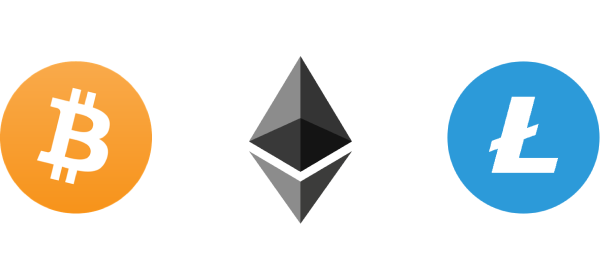Bitcoin, Ethereum, and Litecoin

### Disclaimer

I am not a trader and this blog post is not a financial advice. This is purely introductory knowledge. The conclusion here can be misleading as we analyze the period with immense growth.

### Requirements

For other requirements, see my previous blog post in this series.

### Getting the data

To get the latest data, go to the previous blog post, where I described how to download it using Cryptocompare API. You can also use the data I work within this example.

First, let’s download hourly data for BTC, ETH, and LTC from Coinbase exchange. This time we work with an hourly time interval as it has higher granularity. Cryptocompare API limits response to 2000 samples, which is 2.7 months of data for each coin.

``````import pandas as pd
print('Reading data from %s' % filename)
df.datetime = pd.to_datetime(df.datetime) # change to datetime
df = df.set_index('datetime')
df = df.sort_index() # sort by datetime
print(df.shape)
return df``````

``````df_btc = read_dataset(get_filename('BTC', 'USD', 'Coinbase', 'hour', '2017-12-24'))
df_eth = read_dataset(get_filename('ETH', 'USD', 'Coinbase', 'hour', '2017-12-24'))
df_ltc = read_dataset(get_filename('LTC', 'USD', 'Coinbase', 'hour', '2017-12-24'))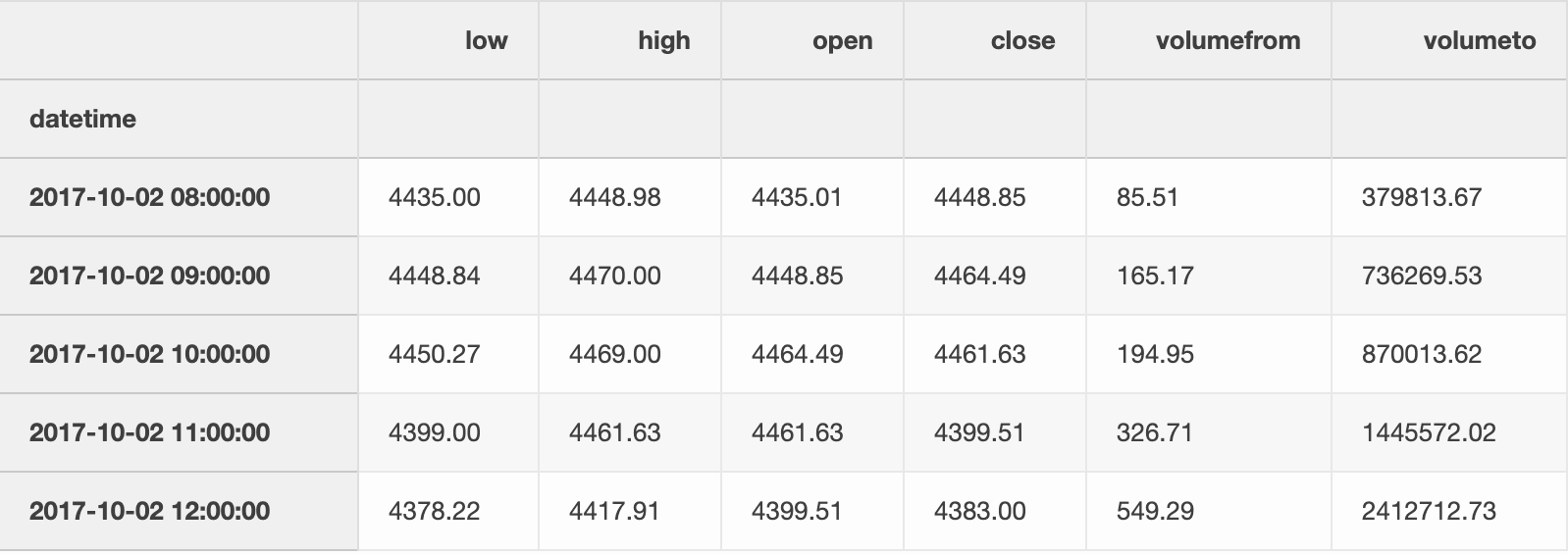Few entries in the dataset.

### Extract closing prices

We are going to analyze closing prices, which are prices at which the hourly period closed. We merge BTC, ETH and LTC closing prices to a Dataframe to make analysis easier.

``````df = pd.DataFrame({'BTC': df_btc.close,
'ETH': df_eth.close,
'LTC': df_ltc.close})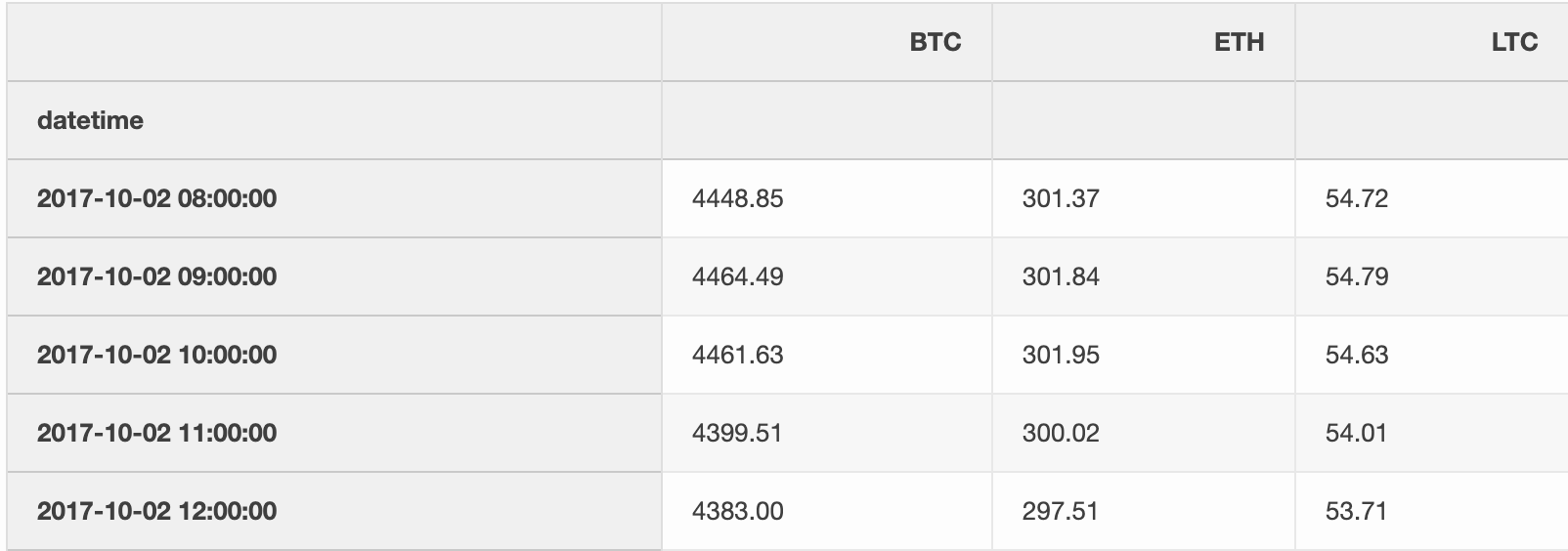### Analysis

#### Basic statistics

In 2.7 months, all three cryptocurrencies fluctuated a lot as you can observe in the table below.

For each coin, we count the number of events and calculate mean, standard deviation, minimum, quartiles, and maximum closing price.

Observations

• The difference between the highest and the lowest BTC price was more than \$15000 in 2.7 months.
• The LTC surged from \$48.61 to \$378.66 at a certain point, which is an increase of 678.98%.
``df.describe()``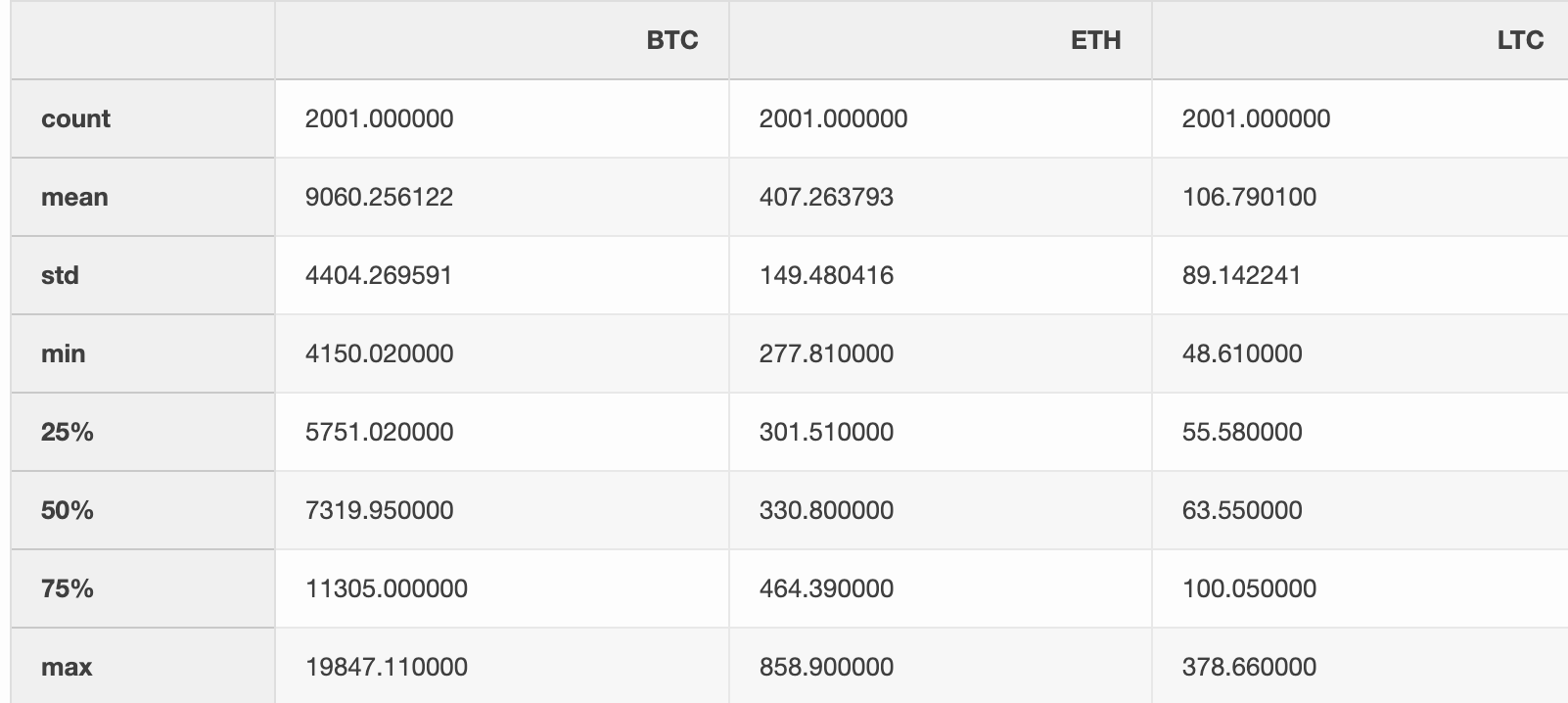### Let’s dive deeper into LTC

We visualize the data in the table above with a box plot. A box plot shows the quartiles of the dataset with points that are determined to be outliers using a method of the inter-quartile range (IQR). In other words, the IQR is the first quartile (25%) subtracted from the third quartile (75%).

On the box plot below, we see that LTC closing hourly price was most of the time between \$50 and \$100 in the last 2.7 months. All values over \$150 are outliers (using IQR). Note that outliers are specific to this data sample.

``````import seaborn as sns
ax = sns.boxplot(data=df['LTC'], orient="h")``````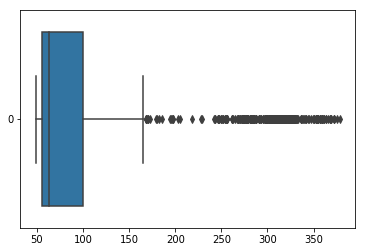LTC Boxplot with closing hourly prices

### Histogram of LTC closing price

Let’s estimate the frequency distribution of LTC closing prices. The histogram shows the number of hours LTC had a certain value.

Observations

• LTC closing price was not over \$100 for many hours.
• it has right-skewed distribution because a natural limit prevents outcomes on one side.
• blue dashed line (median) shows that half of the time closing prices were under \$63.50.
``df['LTC'].hist(bins=30, figsize=(15,10)).axvline(df['LTC'].median(), color='b', linestyle='dashed', linewidth=2)``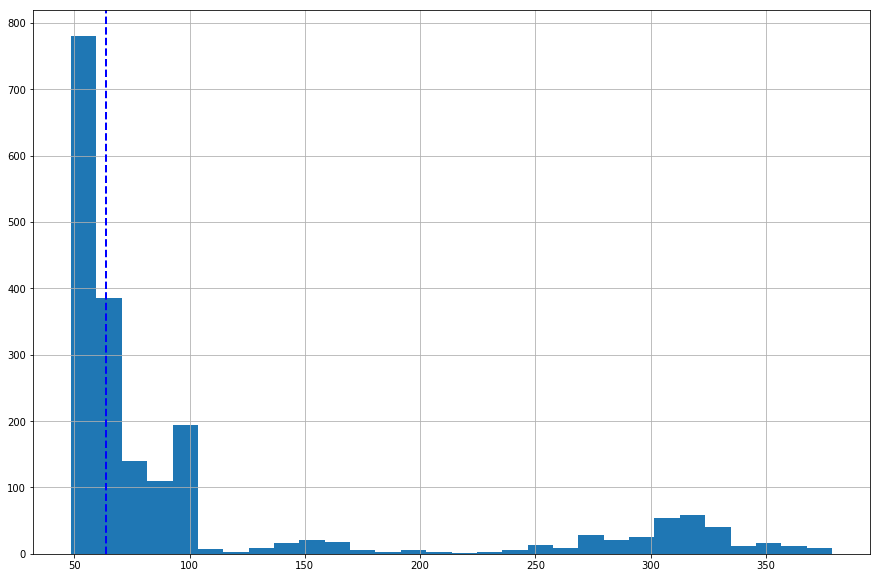Histogram for LTC with the median

### Visualize absolute closing prices

The chart below shows the absolute closing prices. It is not of much use as BTC closing prices are much higher than prices of ETH and LTC.

``df.plot(grid=True, figsize=(15, 10))``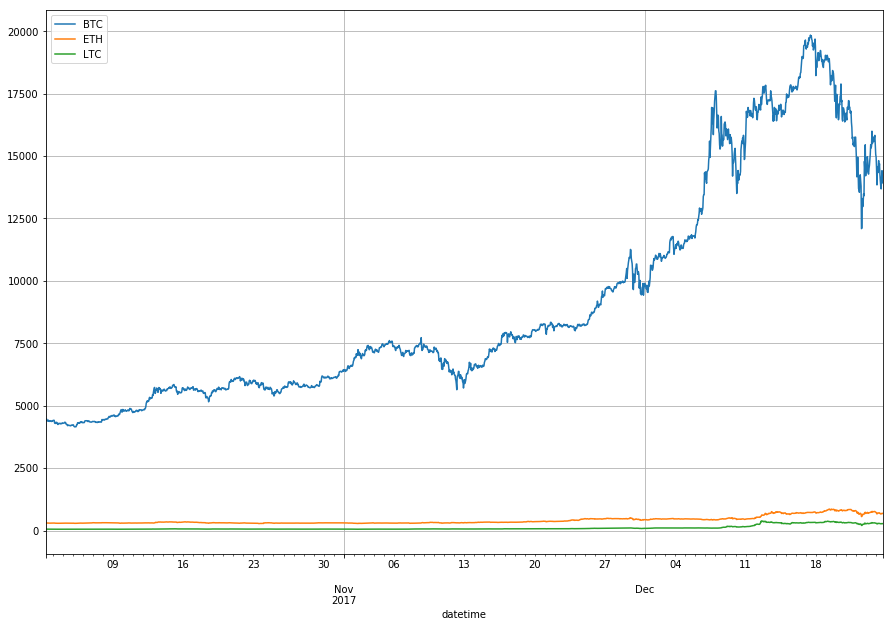Absolute closing price changes of BTC, ETH and LTC

### Visualize relative changes in closing prices

We are interested in a relative change of the price rather than in absolute price, so we use three different y-axis scales.

We see that closing prices move in tandem. When one coin closing price increases so do the other.

``````import matplotlib.pyplot as plt
import numpy as np
fig, ax1 = plt.subplots(figsize=(20, 10))
ax2 = ax1.twinx()
rspine = ax2.spines['right']
rspine.set_position(('axes', 1.15))
ax2.set_frame_on(True)
ax2.patch.set_visible(False)
df['BTC'].plot(ax=ax1, style='b-')
df['ETH'].plot(ax=ax1, style='r-', secondary_y=True)
df['LTC'].plot(ax=ax2, style='g-')
# legend
ax2.legend([ax1.get_lines(),
ax1.right_ax.get_lines(),
ax2.get_lines()],
['BTC', 'ETH', 'LTC'])``````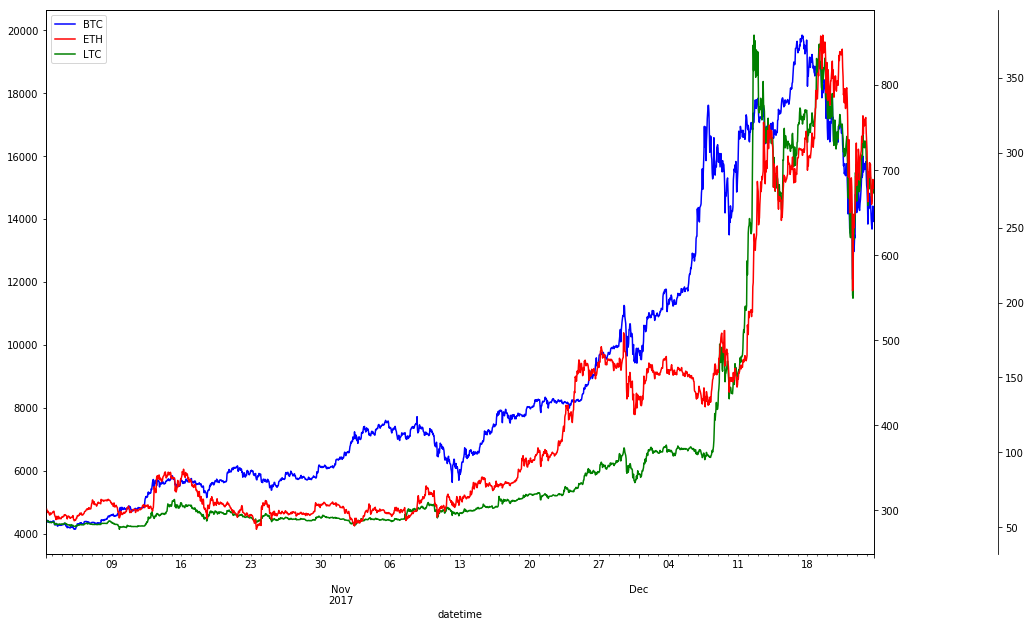Relative closing price changes of BTC, ETH and LTC

### Measure the correlation of closing prices

We calculate the Pearson correlation between the closing prices of BTC, ETH, and LTC. Pearson correlation is a measure of the linear correlation between two variables X and Y. It has a value between +1 and −1, where 1 is the total positive linear correlation, 0 is no linear correlation, and −1 is the total negative linear correlation. The correlation matrix is symmetric so we only show the lower half.

Sifr Data daily updates Pearson correlations for many cryptocurrencies.

Observations

• Closing prices aren’t normalized, see Log Returns, where we normalize closing prices before calculating correlation,
• BTC, ETH and LTC were highly correlated in the past 2 months. This means, when BTC closing price increased, ETH and LTC followed.
• ETH and LTC were even more correlated with 0.9565 Pearson correlation coefficient.
``````import seaborn as sns
import matplotlib.pyplot as plt
# Compute the correlation matrix
corr = df.corr()
# Generate a mask for the upper triangle
# Set up the matplotlib figure
f, ax = plt.subplots(figsize=(10, 10))
# Draw the heatmap with the mask and correct aspect ratio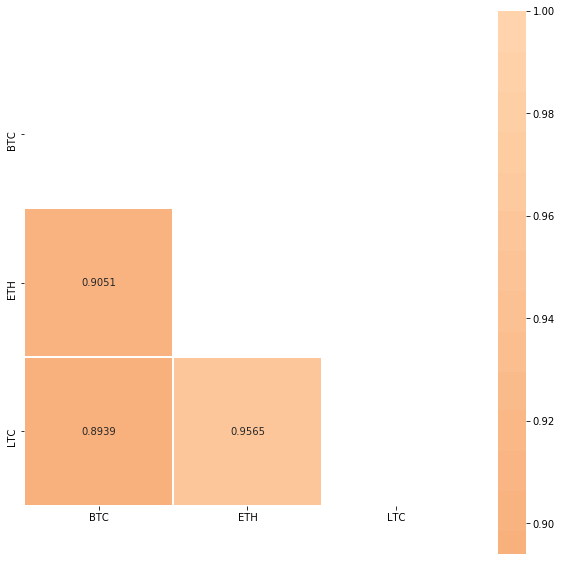Pearson Correlation for BTC, ETH and LTC

Buy and hold is a passive investment strategy in which an investor buys a cryptocurrency and holds it for a long period, regardless of fluctuations in the market.

Let’s analyze returns using the Buy and hold strategy for the past 2.7 months. We calculate the return percentage, where t represents a certain period and price0 is the initial closing price: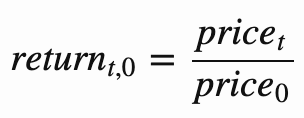``````df_return = df.apply(lambda x: x / x)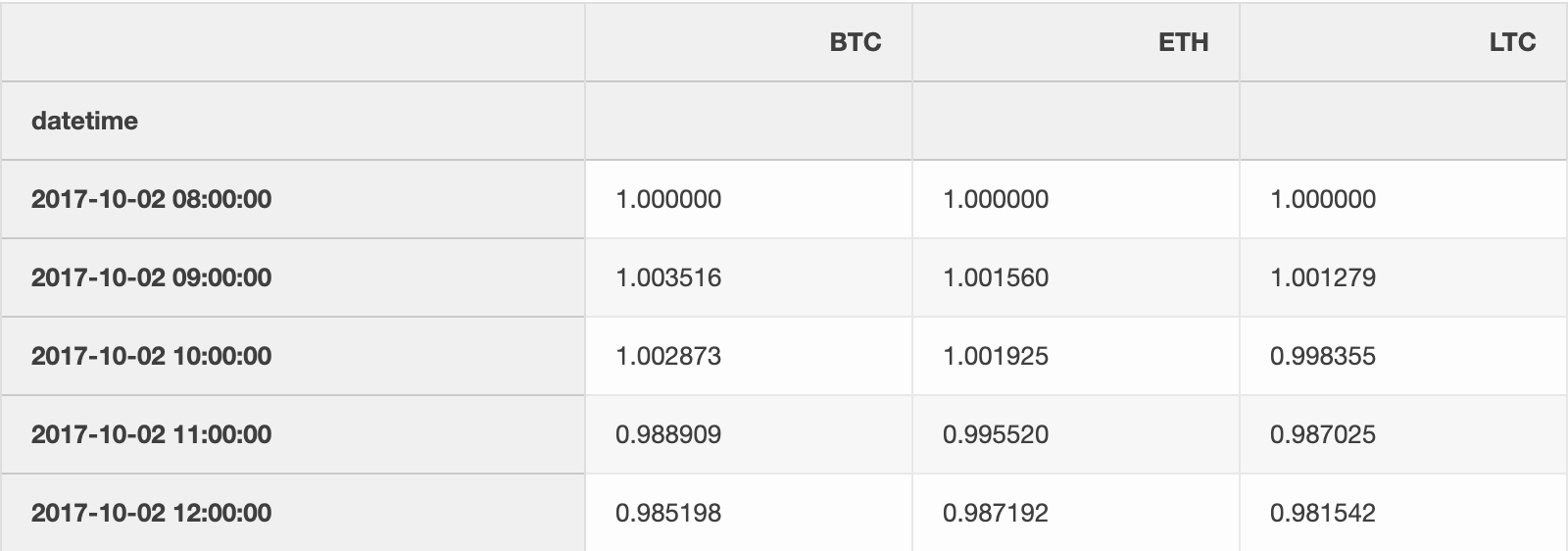### Visualize returns

We show that LTC was the most profitable for the period between October 2, 2017 and December 24, 2017.

``df_return.plot(grid=True, figsize=(15, 10)).axhline(y = 1, color = "black", lw = 2)``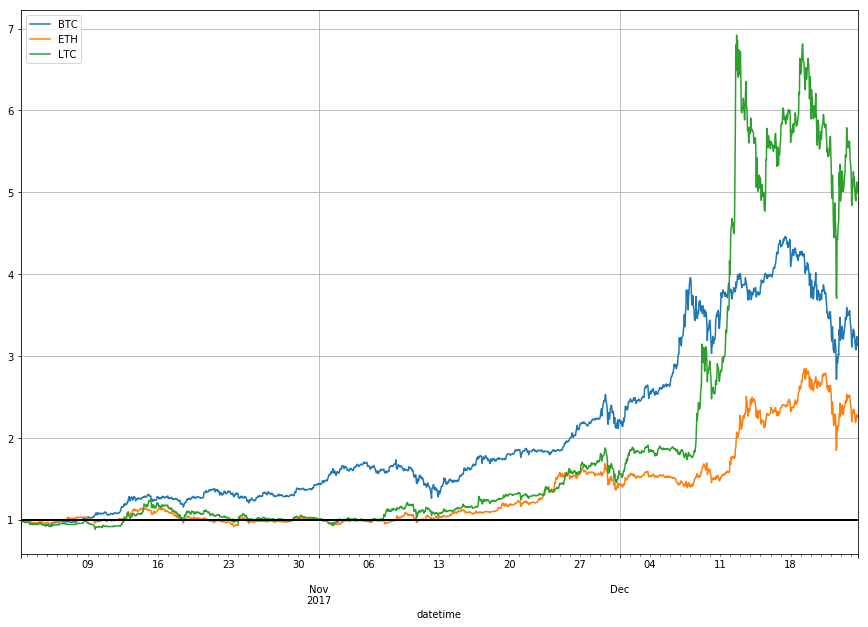#### Conclusion

The cryptocurrencies we analyzed fluctuated a lot but all gained in a given 2.7 months period.

### What about the percentage increase?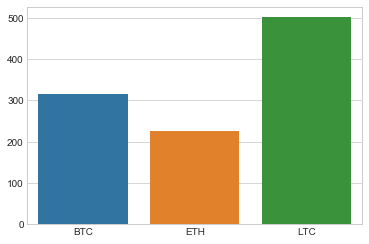The percentage increase for BTC, ETH and LTC in 2.7 months

### How many coins could we buy for \$1000?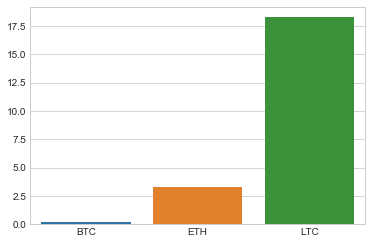The number of coins we could buy with \$1000 a while ago

### How much money would we make?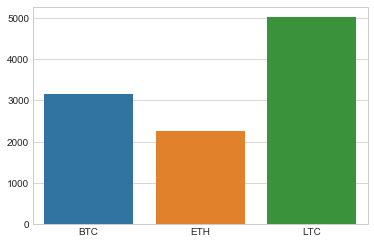## Guide to Python Programming Language

Guide to Python Programming Language

## Python Programming: A Beginner’s Guide

Python is an interpreted, high-level, powerful general-purpose programming language. You may ask, Python’s a snake right? and Why is this programming language named after it?

## Python Hello World Program - Create & Run Your First Python Program in PyCharm

Python Hello World Program - Your first step towards Python world. Learn how to create the Hello World Python program in PyCharm.

## Python Programming Tutorials For Beginners

Python Programming Tutorials For Beginners

## Learn Python Programming

Learn Python Programming